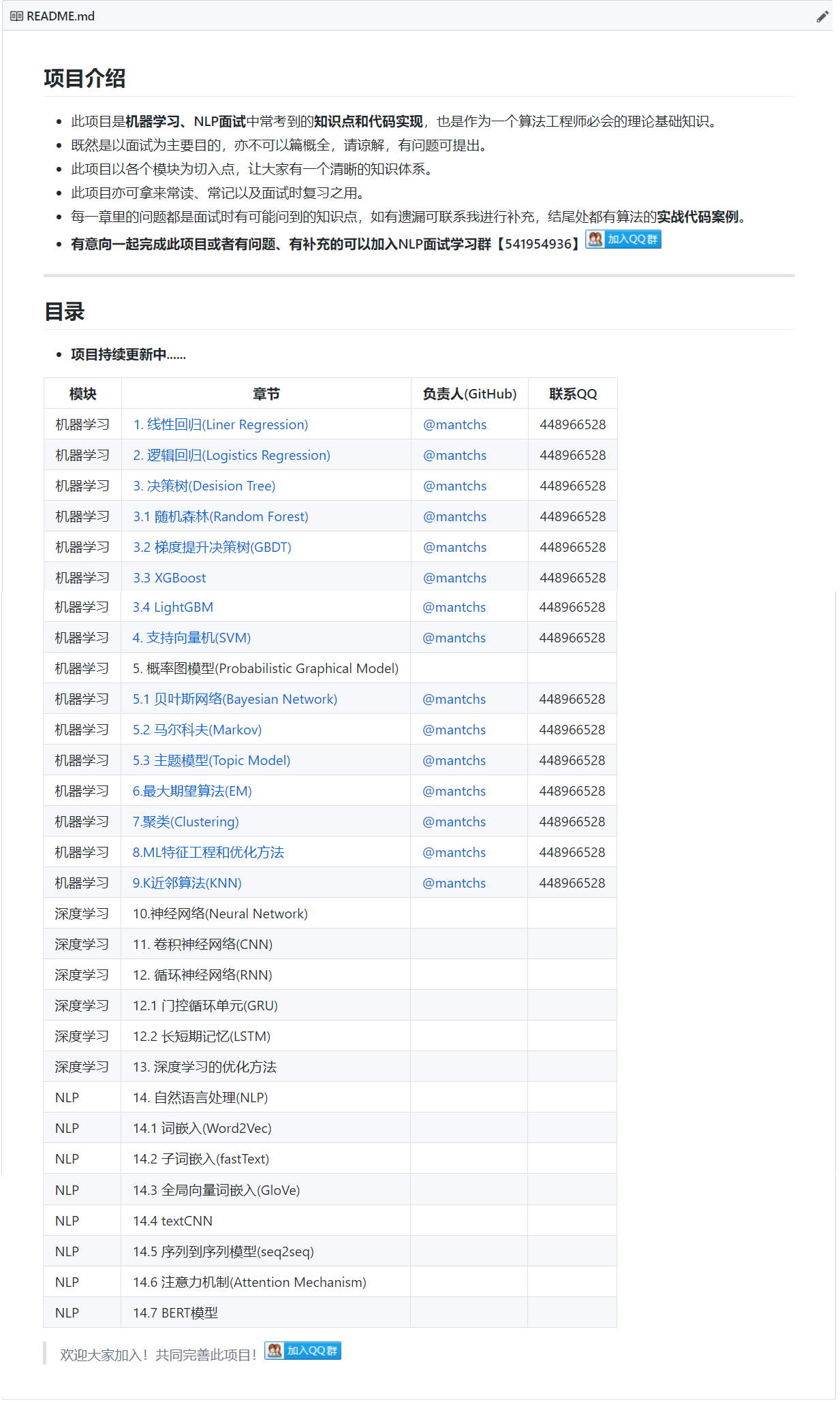# 【NLP】seq2seq

## 1. 什么是seq2seq## 2. 编码器

$$h_t=f(x_t,h_{t-1})$$

$$c=q(h_1,…,h_T)$$

## 3. 解码器

$$P(y_{t^{′}}|y_1,…,y_{t^{′}-1},c)$$

$$s_{t^{′}}=g(y_{t^{′}-1},c,s_{t^{′}-1})$$

## 4. 训练模型

$$P(y_1,…,y_{t^{′}-1}|x_1,…,x_T)=\prod_{t^{′}=1}^{T^{′}}P(y_{t^{′}}|y_1,…,y_{t^{′}-1},x_1,…,x_T)$$

$$=\prod_{t^{′}=1}^{T^{′}}P(y_{t^{′}}|y_1,…,y_{t^{′}-1},c)$$

$$-logP(y_1,…,y_{t^{′}-1}|x_1,…,x_T)=-\sum_{t^{′}=1}^{T^{′}}logP(y_{t^{′}}|y_1,…,y_{t^{′}-1},c)$$

## 5. seq2seq模型预测

### 5.1 贪婪搜索

$$y_{t^{′}}=argmax_{y\in_{}Y}P(y|y_1,…,y_{t^{′}-1},c)$$。由于时间步3所基于的时间步1和2的输出⼦序列由上图中的“A”“B”变为了下图中的“A”“C”，下图中时间步3⽣成各个词的条件概率发⽣了变化。我们选取条件概率最⼤的词“B”。此时时间步4所基于的前3个时间步的输出⼦序列为“A”“C”“B”，与上图中的“A”“B”“C”不同。因此，下图中时间步4⽣成各个词的条件概率也与上图中的不同。我们发现，此时的输出序列“A”“C”“B”“<eos>”的条件概率是0.5 × 0.3 × 0.6 × 0.6 = 0.054，⼤于贪婪搜索得到的输出序列的条件概率。因此，贪婪搜索得到的输出序列“A”“B”“C”“<eos>”并⾮最优输出序列。### 5.2 穷举搜索

10000且T′ = 10时，我们将评估 $10000^{10}=10^{40}$ 个序列：这⼏乎不可能完成。而贪婪搜索的计

### 5.3 束搜索$$\frac{1}{L^{\alpha}}logP(y_1,…,y_L)=\frac{1}{L^{\alpha}}\sum_{t^{′}=1}^{T^{′}}logP(y_{t^{′}}|y_1,…,y_{t^{′}-1},c)$$

## 6. Bleu得分

$$P1= \frac{预测序列中的 1 元词组在标签序列是否存在的个数}{预测序列 1 元词组的个数之和}$$

$$exp(min(0,1-\frac{len_{label}}{len_{pred}}))\prod_{n=1}^{k}p_n^{\frac{1}{2^n}}$$

BLEU为1。

## 7. 代码实现

TensorFlow seq2seq的基本实现